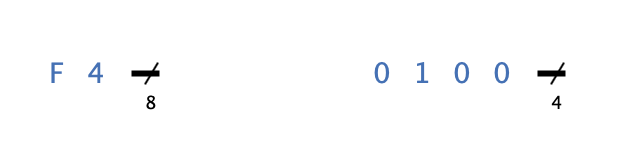## Appearance## Behavior

The constant constantly outputs the configured value at its output.

## Pins

The constant has only one output whose bit width can be configured in the property "Bit Width".

## Properties

Orientation

The direction in which the output pin points.

Bit Width

The number of bits that make up the output signal. If this property is changed so that the number of bits is no longer sufficient to represent the set value in the "Value (decimal)" property, the value is truncated accordingly.

Representation

The way in which the value of the output signal is represented in the representation.

Value (decimal)

The value (in decimal notation) that is output at the output of the constant.